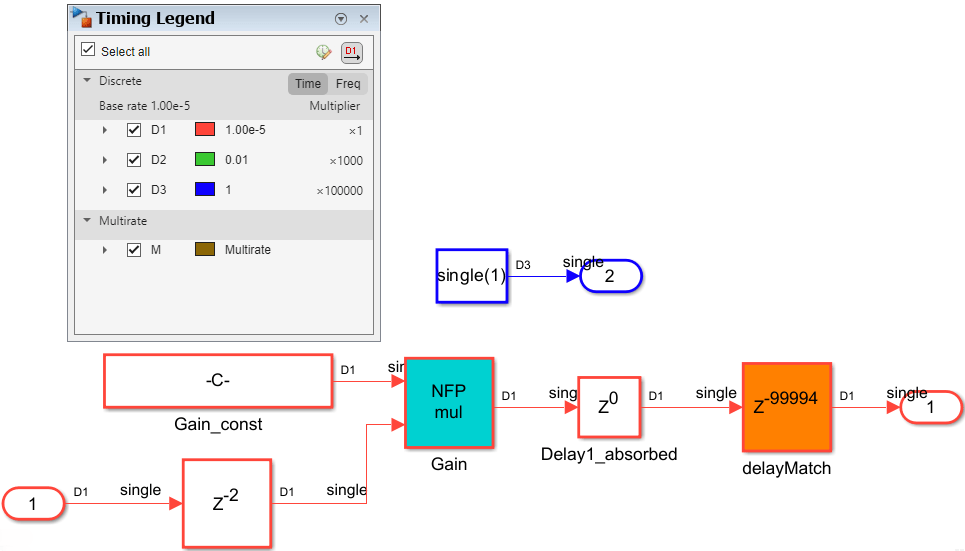# Delay Balancing on Multirate Designs

This example shows how an indiscrete usage of Simulate rates on a multirate design can generate an undesirable HDL code, and provides few recommendations for optimal code generation.

### Introduction

This example model contains 3 subsystems, the first one demonstrates the issue and the others provide practical ways of resolving the issue.

Note in the below design there are two islands of logics, both running at different rates. The rate differential between the two rates is 10E-06, which is a very high number and possibly unrealistic for practical FPGA design. This model has a floating-point Gain block, a multi-cycle operator, in the fast-clock region.Running code generation on this model, we get:The compiled generated model looks as below. Note that the high output latency on the fast clock rate region of the design are added to balance delays across multiple output paths of the system.The high number of registers in the fast clock rate region has an undesired effect post HDL-code generation:

1. Generated HDL files are by itself very large.

2. The large number of pipeline registers will make fitting the design into an FPGA improbable.

The following sections of this document create a general awareness of the resource constraint that multi-rate models can create when used in the presence of multi-cycle operations, and provides a few recommendations for optimum resource usage.

```open_system('hdlcoder_multirate_delaybalancing'); ```### Recommended Guidelines

Your Simulink models might have different clock-rate paths due to different modeling reasons. In the presence of optimizations, like I/O pipelining, distributed pipelining, streaming and/or sharing, or multi-cycle operations, like floating-point IPs, fixed-point math functions like sqrt or divide, pipelines are introduced which are applied at the same rate at which the signal path operates.

Introducing any additional pipelining introduces undesirable latency overhead that needs to be balanced across multiple output paths, operating at different rates. If the ratio difference between the fastest and slowest clock rate in the Simulink model is very large, it causes a large number of registers to be generated in the final HDL code. The HDL files become large and the design may not even fit into an FPGA.

### Recommendation #1: Remove unintentional multirates

There might be an undesirable effect that the rate differential of the model has on the generated HDL code. For instance, in the above model, the sample rate specified on the constant block was not given consideration and set to a value that caused a rate differential of 10E06 with the base model rate. Such a high rate differential appears unintentional.

A recommendation is to change the sample rate of the constant block to run at the same rate as the base model, for such a situation.Running code generation on this model, you get:Note that the output latency numbers have decreased significantly. The compiled generated model looks like the image below.There is no undesirable high number of registers.

### Recommendation #2: Keep rate differential practical

If multi-rate is a desirable property that you need, consider making the rate differential as practical as possible.

For example, if one path of the design running at 'ns' and other path of your design is at 'us' and is a desirable feature of the design, you can still choose to have multi-rate paths in his model with the awareness that delay balancing may cause high number of registers.Running code generation on this model, we get:The compiled generated model looks like the figure below. In the generated model and HDL code, we will have close to 1000 registers in the fast clock rate output path. The additional cost of registers is not unusual for control logics that are running 1000x faster than the system. It is important to be aware of the hardware resource constraints for such a model.To optimize on the total number of registers in FPGA, you can also use the model parameter Map pipeline delays to RAM. Doing this can tradeoff RAM resources to save on logic area.

>> hdlset_param(gcs, 'MapPipelineDelaysToRAM', 'on');